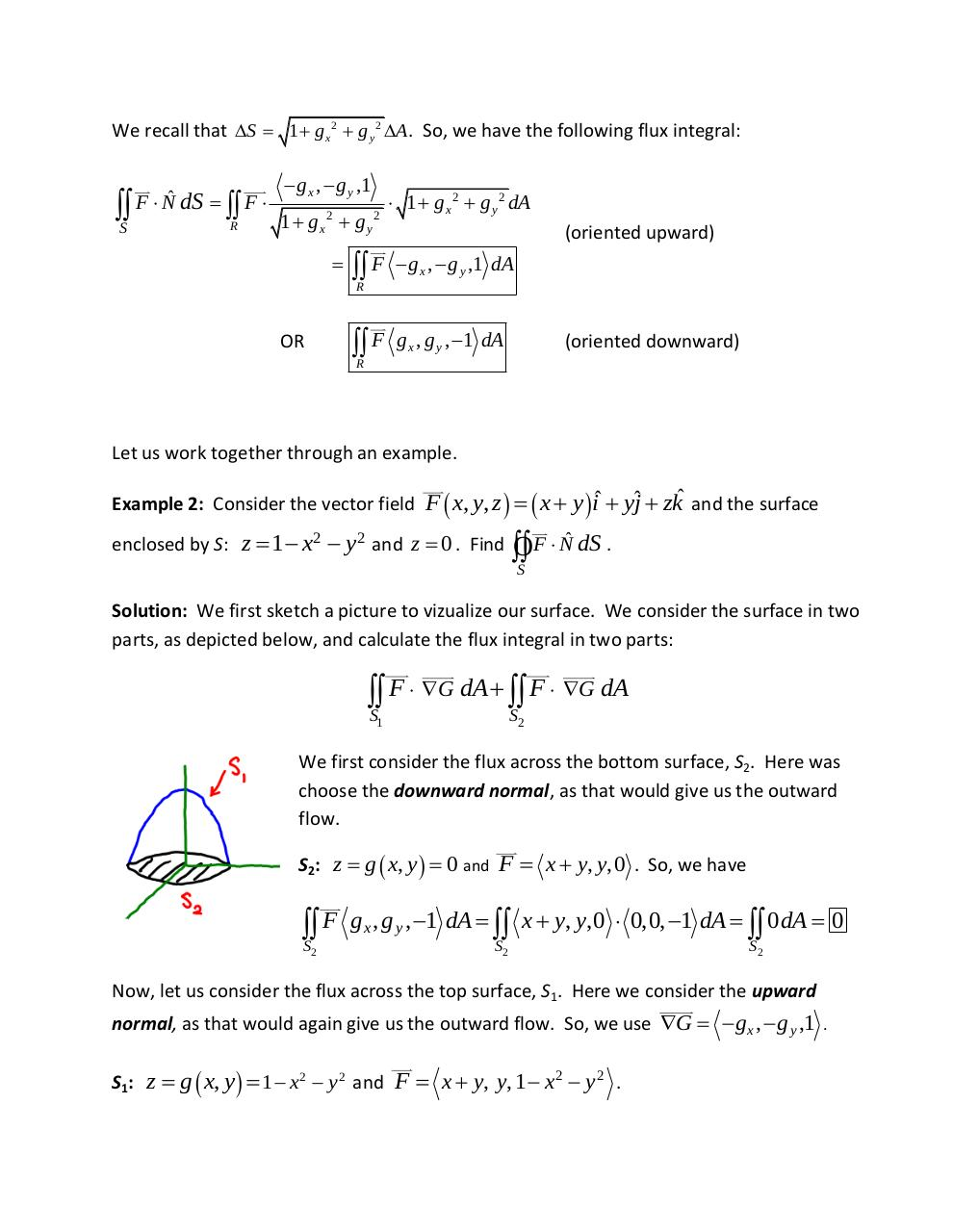# Lesson 10 6 Surface Integrals.pdfPage 1 2 3 4 5 6 7

#### Text preview

We recall that S  1  g x 2  g y 2 A . So, we have the following flux integral:

S

F  Nˆ dS   F 
R

 g x ,  g y ,1
1  gx  g y
2

OR

2

 1  g x 2  g y 2 dA
(oriented upward)

R F

 g x ,  g y ,1 dA

R F

g x , g y , 1 dA

(oriented downward)

Let us work together through an example.
Example 2: Consider the vector field F  x, y, z    x  y  iˆ  yjˆ  zkˆ and the surface
enclosed by S: z  1  x2  y 2 and z  0 . Find

OF  Nˆ dS .

S

Solution: We first sketch a picture to vizualize our surface. We consider the surface in two
parts, as depicted below, and calculate the flux integral in two parts:

S F  G dA  S F  G dA
1

2

We first consider the flux across the bottom surface, S2. Here was
choose the downward normal, as that would give us the outward
flow.
S2: z  g  x, y   0 and F  x  y, y,0 . So, we have

S F
2

g x , g y , 1 dA   x  y, y,0  0,0, 1 dA   0 dA  0
S2

S2

Now, let us consider the flux across the top surface, S1. Here we consider the upward
normal, as that would again give us the outward flow. So, we use G   g x ,  g y ,1 .
S1: z  g  x, y   1  x2  y 2 and F  x  y, y, 1  x2  y 2 .